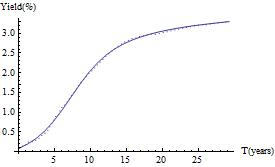# A Young Quant’s Illustrated Primer: the SABR Model

## What is the SABR model?

The SABR model (pronounced as in light saber) is a popular stochastic volatility model. A somewhat more general version than the one we discuss here was introduced by P. Hagan and co-authors in 2002, with applications to interest rate-related derivatives. The model became popular because a (quite difficult) small-time analysis resulted in some easy-to-apply, approximate, “smile” formulas that could fit the authors’ target markets.

While equity applications are rare, the model makes mathematical sense for equities, where the stochastic process pair is$\{S_t,\sigma_t\}$. Here$S_t \ge 0$ is a stock price and$\sigma_t > 0$ is the associated stochastic volatility. Using that equity language, the SABR model, as an SDE (stochastic differential equation) system, reads$\displaystyle \left \{ \begin{array}{l}dS_t = \sigma_t S_t^{\beta} dB_t, \quad (t < \tau_0), \\d \sigma_t = \nu \, \sigma_t \, dW_t, \quad dB_t \, dW_t = \rho \, dt. \end{array}\right. \quad\quad\quad\quad\quad (1)$

In equation (1),$\beta$ is any real number, and$\nu > 0$ is the “volatility of volatility”. The latter can be set equal to one without loss of generality, so we’ll do so for the remainder of this post. Although any real$\beta$ makes mathematical sense, fits to market data will almost always have$-\infty < \beta \le 1$, so we’ll stick to that range also. Also$\rho \in (-1,1)$ is a correlation parameter. Finally,$\tau_0$ is the first time, if any, that the stock price hits 0.

## Boundary conditions

The stock price can hit 0 with a positive probability whenever$\beta$ is strictly less than 1. What happens after that requires a boundary condition. Of course, for equities, to avoid an arbitrage opportunity, once a stock price hits zero, it must stay there (unless the company is recapitalized). So, an additional specification of the equity version of the model is that$S_t = 0$ for all$t \ge \tau_0$.

For the original fixed income applications, the underlying security is a (forward) interest rate and other boundary conditions are possible.

## Exact solutions

By a “solution” to equation (1), I mean a solution for the associated transition probability density. Once you have that, option values, for example, are found by an integration. Exact solutions are known for the following special cases:

• Normal SABR model:$\beta = 0$ with$\rho = 0.$
• Lognormal SABR model:$\beta = 1$ with any$\rho.$

## McKean’s formula

The Normal SABR model solution is found by adapting a famous formula for the transition density found by Henry P. McKean in 1970. For that case, it makes more sense to switch gears slightly in the notation and use$X_t$ instead of$S_t$, now$X_t$ the location of a “particle” which can hit 0 and keep on going to arbitrarily negative values. With that new notation, the Normal SABR model process is:$\displaystyle \left \{ \begin{array}{l}dX_t = \sigma_t dB_t, \\d \sigma_t = \sigma_t \, dW_t. \end{array}\right. \quad\quad\quad\quad (2)$

Now$B_t$ and$W_t$ are two independent Brownian motions. Equation (2) also describes a generalized type of Brownian motion, namely Brownian motion on a two-dimensional hyperbolic space.

Suppose we start the particle at$X_0 = x_0 = 0$ and$\sigma_0 = 1$. Then, the probability of finding the particle at subsequent times is given by Mckean’s formula for$G(t,X,\sigma|x_0,\sigma_0)$. If we let t range from 0.01 to 1, we can plot McKean’s formula to findÂ the following evolution:Evolution of McKean formula probability density for the Normal SABR model

If you watch closely, you’ll see that the probability density starts with a spike near$(X,\sigma) = (0,1)$ and then spreads out in the X direction. In the$\sigma$ direction, mass begins to accumulate near$\sigma \rightarrow 0.$ That’s due to lack of mean reversion in the volatility process and is an ‘unphysical’ aspect of the model.

Now that we’ve talked about it so much, you may be curious as to what this mysterious formula looks like. Here it is:$\displaystyle G(t,X,Y|x,y) = \frac{e^{-t/8}}{2 \, Y^2 \, (\pi t)^{3/2}} \int_d^{\infty} \frac{s \, e^{-s^2/(2 t)}}{\sqrt{\cosh s - \cosh d}} \, ds,$$\displaystyle \mbox{where} \quad d = \cosh^{-1}z, \quad \mbox{using} \quad z = 1 + \frac{(X-x)^2 + (Y-y)^2}{2 y Y}$

To adapt the McKean solution for an equity model, you can use the well-known “method of images”. The method will ensure that the associated equity process$S_t$, which starts at$S_0 > 0$, is absorbed should it hit$S=0.$

(There’s much more to the model than we have touched upon, and we’ll certainly return to it in future blog posts. This post has been adapted from material in Ch. 8 (“A Closer Look at the SABR Model”) from “Option Valuation under Stochastic Volatility II”).

Animation code

# Started adding book codes from ‘Option Valuation under Stochastic Volatility II’

I have started adding book codes to this site. Almost all will be Mathematica notebooks (ending in .nb) , although there will be some C/C++. This may be a slow process since most files will require some clean-up before posting.

One file has been added so far: from Ch. 1: CIRJumpStart.nb, which was used to generate Fig. 1.6 in the book and below:Fig. 1.6. CIR Bond Process with a Delayed Jump-start.

The figure shows a fit of the CIR bond process with a delayed jump-start (solid curve) to the US Treasury Zero Coupon yield curve (dashed curve), using Feb. 10, 2012 data. The delayed jump-start is/was a mechanism to model the end of the ZIRP (Zero Interest Rate Policy) regime. Details and related models are found in the book Chapter 1: “Slow Reflection, Jump-returns, and Short-term Interest Rates”.

The code file location is the Latest Book link (Just click on ‘Selected Codes’)

I will probably just work my way slowly through the book, but if you are a reader and particularly want to see certain book codes, please let me know by a comment or email. I will try to accommodate if possible.